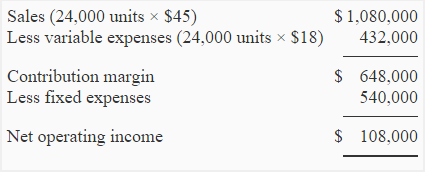# Problem-4 (CM ratio, degree of operating leverage, break-even point)

ECG company sells lightweight tables. One table is sold for \$45. Variable and fixed expenses data is given below:

• Variable expenses per unit: \$18
• Fixed expenses per year: \$540,000

Required:

1. Compute contribution margin ratio (CM ratio).
2. Compute break-even point in dollars using CM ratio computed in part 1.
3. Using contribution margin ratio calculate increase in net operating income if sales are increased by \$135,000.
4. During the last year, ECG company sold 24,000 lightweight tables.
(a). Compute the degree of operating leverage at the last year’s level of sales.
(b). If ECG company manages to increase the sales by 15% next year, by how much should net operating income increase? (Use degree of operating leverage for your answer).

## Solution:

### (1) Calculation of CM ratio:

Contribution margin = Selling price – Variable expenses

= \$45 – \$18

= \$27

Contribution margin ratio (CM ratio) = Contribution margin/Sales

= \$27/\$45

= 0.60 or 60%

### (2) Calculation of break-even point:

Break-even point in sales = Fixed cost/CM ratio

= \$540,000/0.60

= \$900,000

\$135,000 × 0.6

\$81,000

### (4) Degree of operating leverage:(a). Degree of operating leverage = Contribution margin/Net operating income

= \$648,000 / \$108,000

= 6

(b). The degree of operating leverage is 6. It means if sales are increased by 15%, there will be a 90% increase in net operating income as computed below:

15% × 6 = 90%

A D V E R T I S E M E N T
3 Comments on Problem-4 (CM ratio, degree of operating leverage, break-even point)
1.eunju kim

it’s really useful and understandible to use…

thank you !!

2.Ilyas

People, can you help? How to analyze the degree of operating leverage when NI is negative? Does it simply mean that the measure is useless in this like situations?

3.Monirul Islam

Good